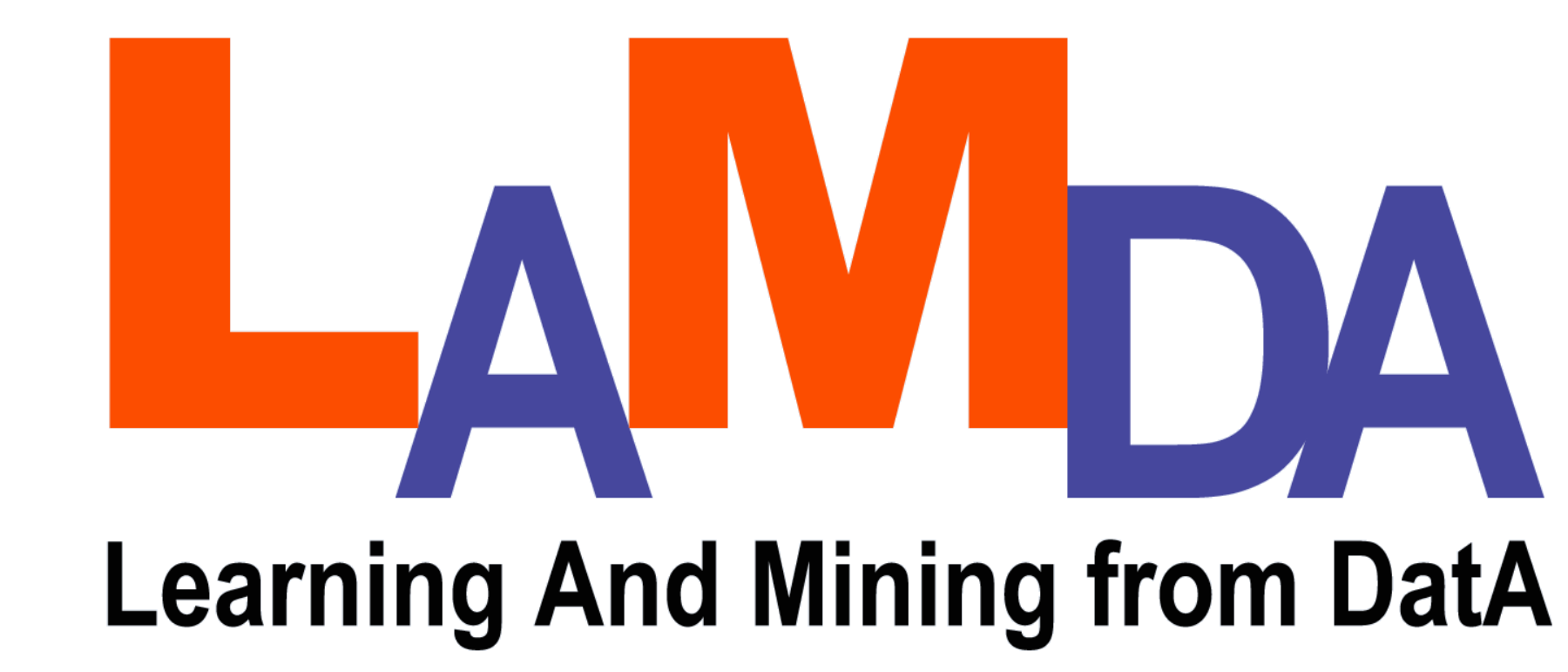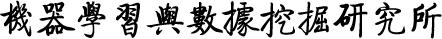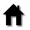Home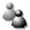People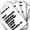Publication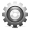Applications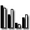Data & Code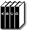Library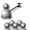Seminar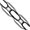Link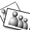Album

Search LAMDA
»

# Data & Code

The code or data listed below were developed or collected by LAMDA members. They are shared here for expediating the communication of research results among scientific communities. They can be freely used at your own risk, given that the contributions of LAMDA are appropriatedly cited or acknowledged in your publications.Note: They can only be used for academic purpose. For other purposes, please contact with Prof. Zhi-Hua Zhou.

[Data] [Code/Demo]

# Data¶

• RFID Data for Feature Evolvable Streaming Learning.

• MDDM Data for multi-label dimensionality reduction.

• MILWEB Data for Multi-Instance Learning Based Web Index Recommendation.

• SGBDota Data for the PCES (Positive Concept Expansion with Single snapshot) problem.

# Code¶

• AcMR The package includes the python code of the AcMR algorithm for the rapid performance improvement problem in model reuse studies.

• AdaTTL The package includes the MATLAB code of the Adaptively Trimming off Tail Labels (AdaTTL) which aims to trim tail labels and facilitate fast prediction and compact models in large-scale multi-label learning.

• AKPCA AKPCA is a package for adaptive kernel selection for kernel principal component analysis. This package includes the MATLAB implementation of AKPCA, which can effectively learn the kernels when the class labels of the training examples are not available.

• AUDI AUDI is a package for multi-label active learning. This package includes the MATLAB implementation of AUDI, which queries instance-label pairs based on both uncertainty and diversity.

• AURO AURO is a package for multi-label active learning with a novel query type. This package includes the MATLAB implementation of AURO, which iteratively selects a triplet consisting of one instance and two labels, and queries the relevance ordering of the label pairs on the instance.

• AutoSSL The package includes the matlab code of the AutoSSL algorithm for automatically selecting semi-supervised learning algorithms and hyperparameters. You will find an example of using this code in the 'Ex_autossl.m' function.

• BAMIC BAMIC is a package for multi-instance clustering. This package includes the MATLAB implementation of B AMIC , which is designed to deal with unsupervised multi-label learning problems. It is particularly useful when real-world objects are represented under multi-instance setting while the labels of these objects are unknown.

• BPMIP BPMIP is a package for training multi-instance BP neural networks. The package includes the MATLAB code of the algorithm BP-MIP. It is very easy to implement BP-MIP-DD and BP-MIP-PCA based on this package. Actually, running Diverse Density at first and then using the learned scales to rescale the attributes before presenting the data to BPMIP, you get BP-MIP-DD; running principal component analysis (PCA) at first and then presenting the projected data to BP-MIP, you get BP-MIP-PCA

• BPMLL BPMLL is a package for training multi-label BP neural networks. The package includes the MATLAB code of the algorithm BP-MLL, which is designed to deal with multi-label learning. It is in particular useful when a real-world object is associated with multiple labels simultaneously.

• C4.5Rule-PANE C4.5Rule-PANE is a rule learning method which could generate accurate and comprehensible symbolic rules, through regarding a neural network ensemble as a pre-process of a rule inducer.

• CAPO This package provides the demo implementation of CAPO (i.e. Classifier Adaptation for Performance measure Optimization), which takes a classifier adaptation approach to optimize the concerned performance measure (including AUC and all the performance measures based on the contingency table like F1-score and PRBEP). A readme file is included in the package.

• CCE CCE is a multi-instance learning method solving multi-instance problems through adapting multi-instance representation to single-instance algorithms, which is quite different from existing multi-instance learning algorithms which attempt to adapt single-instance algorithms to multi-instance representation.

• CEAL CEAL is a package for cost-effective active learning in crowdsourcing setting, where multiple labelers are available to offer diverse qualities of labeling with different costs.

• cisLDM cisLDM is a package which tries to optimize the margin distribution on both labeled and unlabeled data when minimizing the worst-case total-cost and the mean total-cost simultaneously according to the cost interval. The package includes the MATLAB code of the algorithm cisLDM and one example data set.

• CISVM CISVM is a package for learning with cost intervals. It can be used when the precise cost information is not known while cost intervals are available.

• ClustererEnsemble ClustererEnsemble is a package containing methods for building ensembles of clusterers. In particular, ensembles of k -means clusterings are constructed with voting, weighted voting, selective voting, and selective weighted voting.

• CS4VM CS4VM is a package for efficient cost-sensitive semi-supervised learning. The package includes the MATLAB code of the algorithm CS4VM.

• CS-LapSVM This package includes the MATLAB codes of CS-LapSVM, which is a cost-sensitive extension of Laplacian support vector machines.

• CSNN This package contains 6 algorithms for training cost-sensitive neural networks. They are over-sampling, under-sampling, threshold-moving, SMOTE and two ensemble methods, i.e. hard-ensemble and soft-ensemble.

• CoForest CoForest is a semi-supervised algorithm, which exploits the power of ensemble learning and large amount of unlabeled data available to produce hypothesis with better performance.

• COREG COREG is a co-training style semi-supervised regression algorithm, which employs two k-NN regressors using different distance metrics to select the most confidently labeled unlabeled examples for each other.

• CoTrade The package includes the MATLAB code of COTRADE, which is designed for enhancing traditional co-training algorithm by incorporating data editing techniques. A Readme file and with the sample data are included in the package.

• DDT The package includes the MATLAB code of the image co-localization methods DDT and DDT+, which are efficient and effective co-localization algorithms based on deep pre-trained CNN models.

• DeepMIML This is the official clone for the implementation of DeepMIML Network. It is a deep model for multi-instance multi-label learning.

• DMNL This package includes the MATLAB codes of a DMNL, which discovers multiple novel labels in multi-instance multi-label learning. Two demo programs are included in the package, one is on a synthetic dataset, and the other is on MSRCv2 data.

• DREP This package provides an implementation of the DREP method (i.e., Diversity Regularized Ensemble Pruning), which explicitly exploit diversity regularization in ensemble pruning.

• DRM This package provides an implementation of the DRM method (i.e., Diversity Regularized Machine), which trains multiple SVM with a diversity constraint.

• DSML DSML is a package for dual set multi-label learning. This package includes the MATLAB implementation of DSML, which is a boosting-like algorithm with model-reuse and distribution adjusting mechanisms.

• EasyEnsemble EasyEnsemble and BalanceCascade are two class-imbalance learning methods. They can adaptively exploit the majority class examples, avoiding important majority class examples to be ignored by common under-sampling while maintaining the fast training speed of under-sampling.

• EForest eForest: A python implementation of encoderForest (eForest), a forest based autoencoder.

• EnMIMLNNmetric This package includes the MATLAB code of the EnMIMLNNmetric. It is a novel ensemble MIML learning framework by combining the advantage of three kinds of Hausdorff distance metrics.

• Demo of FANNC and FANRE FANNC is a fast neural classifier, and FANRE is a fast neural regressor. Both are developed based on Adaptive Resonance Theory and Field Theory. Prominent characteristics of these neural networks mainly include: they do not require the user to setup the number of hidden units; they only scan the training set once; they are incremental learning algorithms that can be used in online learning environments; etc.

• Demo of Wu&Zhou's Face Detector Wu&Zhou-FaceDetector is a demo for an efficient face candidates selector proposed for face detection tasks in still gray-level images.

• FASBIR FASBIR is a variant of Bagging algorithm, whose purpose is to improve accuracy of local learners, such as kNN, through multi-model perturbing ensemble.

• FESL The package includes the MATLAB code of the FESL (Feature Evolvable Streaming Learning) which focuses on the learning with feature evolvable streams. The package includes two proposed approaches FESL-c and FESL-s as well as three baseline methods.

• GASEN GASEN is a selective ensemble method using genetic algorithm to help select a subset of neural networks (or other learners, with appropriate modification) to compose an ensemble, which is better than directly ensembling all the neural networks available.

• gcForest A python 2.7 implementation of gcForest.

• gcForestCS A demo implementation of gcForest library as well as some demo client scripts to demostrate how to use the code. The implementation is flexible enough for modifying the model or fit your own datasets.

• Glocal GLOCAL is a package for Multi-Label Learning with Global and Local Label Correlation, which is designed to explore both the global and local label correlations for multi-label learning, and can handle missing labels. This package includes the MATLAB implementation of GLOCAL.

• InsDif INSDIF is a package for learning multi-label classifiers through instance differentiation. The package includes the MATLAB code of the algorithm I NS D IF , which is designed to deal with multi-label learning. It is in particular useful when a real-world object is associated with multiple labels simultaneously.

• ISD ISD (Instance Specific Distance) is a package for learning instance specific distance functions. By using this package, you are able to assign instance specific distances for each labeled examples as well as unlabeled examples. The key of ISD learning is metric propagation.

• KISVM KISVM is a package for locating the key instances (e.g., ROIs in image retrieval) in multi-instance learning. There are two versions, i.e., Bag KISVM and Instance KISVM.

• KISAR KISAR is a package towards discovering what patterns trigger what labels with MIML(Multi-Instance Multi-Label learning). The package includes the MATLAB code of the algorithm KISAR.

• LACU-SVM LACU-SVM is a package towards recognizing augmented classes by exploiting unlabeled data. The package includes the MATLAB and C++ code of the algorithm LACU-SVM.

• LALOT LALOT is a package to implement label distribution learning by optimal transport (LALOT) algorithm. The package includes the MATLAB code of the algorithm LALOT.

• LEAD LEAD is a package towards making inappropriate graphs never deteriorate the performance. This package includes the MATLAB codes of a large margin separation method LEAD for safe graph-based semi-supervised learning.

• LDM LDM is a package which tries to achieve strong generalization performance by optimizing the margin distribution. The package includes the MATLAB code of the algorithm LDM and two example data sets.

• LGMMC LGMMC is a package for maximum margin based clustering. The package includes the MATLAB code of the algorithm LG-MMC. There are two kinds of codes. One is used for small data with linear and rbf kernel. The other is used for large scale data with linear kernel only. It is very easy to implement LG-MMC in these two setting. You just need to read/run experiment.m file in the package. Moreover, we include two simple data sets as examples.

• LIoIS The package includes the MATLAB code of Learning from Incomplete and Inaccurate Supervision (LIoIS).

• LMLL-POP The package includes the MATLAB code of the POP algorithm which towards learning compact models for large-scale multi-label learning data with jointly label parameter optimisation and feature parameter optimisation.

• LS-ILDA LS-ILDA is an incremental version of the least-square linear discriminant analysis. When a new instance comes, instead of computing discriminant componenet from scratch, LS-ILDA updates the components obtained in the last step. It has two updating mode, corresponding to whether the dimension is larger than the number of instances.

• M3LDA This package provides the demo implementation of M3LDA (i.e. Multi-Modal Multi-Instance Multi-Label LDA).

• M3MIML M3MIML is a package for learning from multi-instance multi-label examples by maximum margin strategy. The package includes the MATLAB code of the algorithm M3MIML, which is designed to deal with multi-instance multi-label learning. It is in particular useful when a real-world object is represented by multiple instances and associated with multiple labels simultaneously.

• MAHR MAHR is a package for multi-label hypothesis reuse. MAHR exploit label relationship by reusing hypothesis across labels. The package includes the MATLAB code of the MAHR algorithm.

• Maxide Maxide is a matrix completion method when side information is available.

• mcKLR mcKLR is a package for multi-class cost-sensitive learning. It has been applied to face recognition with success in our CVPR'08 paper. In that paper we argue that face recognition is inherently a task involving unequal misclassification costs, and therefore we should try to minimize the costs instead of minimizing the number of mistakes, yet almost all previous face recognition research focus only on minimizing the number of mistakes! The mcKLR method, however, can also be applied to other tasks which involve multi-class cost-sensitive learning.

• mcODM mcODM is a package which tries to achieve strong generalization performance by optimizing the margin distribution for multi-class learning. The package includes the C++ source code and the MATLAB interface of the algorithm mcODM and a demo with some example data sets.

• MDDM MDDM is a package for multi-label dimensionality reduction. It can be used to reduce the dimensionality of high-dimensional multi-label data.

• meanS3VM MeanS3VM is a package for efficient semi-supervised learning using label mean. The package includes the MATLAB code of the algorithm MeanS3VM. Two algorithms are included. One is MeanS3VM-iter, while the other is MeanS3VM-mkl.

• mGBDT This is the official clone for the implementation of mGBDT.

• MIEN This is the official clone for the implementation of MIEN.

• MIKI The package includes the MATLAB code of the MIKI (Multi-Instance Learning with Key Instance Shift) algorithm which focuses on handling the setting when Multi-instance learning encounters with key (positive) instance shift. You will find an example of using this code in the 'example.m' function. The example data is 20newsgroup dataset comp_gra_ibm.

• miVLAD/miFV The package includes the MATLAB code of the multi-instance learning algorithms miVLAD and miFV, which are efficient and scalable MIL algorithms. A Readme file and some example files are included in the package.

• miGraph The package includes the MATLAB code of the multi-instance learning algorithm miGraph, which does not assume the instances as i.i.d. samples. A Readme file and some example files are included in the package.

• MI-Ensemble This toolbox contains re-implementations of four different multi-instance learners, i.e. Diverse Density, Citation-kNN, Iterated-discrim APR, and EM-DD. Ensembles of these single multi-instance learners can be built with this toolbox.

• MIL_BG The package includes the MATLAB codes of the nine image bag generators algorithms for multi-instance learning.

• MIML This page provides a collection of MATLAB codes of different MIML related algorithms, including: MIMLBoost, MIMLSVM, MIMLSVMmi, MIMLNN, D-MIMLSVM, InsDif and SubCod. For each algorithm, there is a readme file with a simple example to show how to use it.

• MIMLdros MIMLdros includes the MATLAB codes of MIMLSVM+ and E-MIMLSVM+ which are MIML algorithms that have been applied to drosophila gene expression pattern annotation. The package contains a Readme file which explains how to use it.

• MIMLBOOST & MIMLSVM The package includes the MATLAB code of algorithms MIMLBOOST and MIMLSVM , both of which are designed to deal with multi-instance multi-label learning. It is in particular useful when a real-world object is associated with multiple instances as well as multiple labels simultaneously.

• MIMLfast This package includes the MATLAB codes of MIMLfast, which is a fast algorithm for multi-instance multi-label learning.

• MIMLmix MIMLmix package implements MIMLmix model for Multi-view Multi-instance Multi-label learning.

• MIMLWEL This package includes the MATLAB code of the multi-instance multi-label learning algorithm MIMLWEL.

• MissSVM MissSVM is a package for solving multi-instance learning problems using semi-supervised support vector machines. The purpose of MissSVM is to show that if the assumption of i.i.d. instances were taken, multi-instance learning can be viewed as a special case of semi-supervised learning, and the field of multi-instance learning might be merged into the field of semi-supervised learning. Thus, future multi-instance learning research should assume only i.i.d. bags and avoid the assumption of i.i.d. instances

• MLCCE MLCCE is a package for utilizing the label relationship in multi-label learning, which overcomes the drawback that appropriate label learning order is hard to obtain when utilizing the label relationship for training. This package includes the MATLAB codes of the CCE method.

• MLKNN ML-KNN is a package for learning multi-label k -nearest neighbor classifiers. The package includes the MATLAB code of the algorithm ML-KNN, which is designed to deal with multi-label learning. It is in particular useful when a real-world object is associated with multiple labels simultaneously

• ML-LOC ML-LOC is a package for multi-label learning by exploiting label correlations locally.

• mlODM mlODM is a package which tries to achieve strong generalization performance by optimizing the margin distribution for multi-label learning. The package includes MATLAB code of the algorithm mlODM and a demo with example data set.

• MOMS This package includes the JAVA code of the MOMS algorithm for maximizing monotone k-submodular functions under a size constraint.

• MTI-Diversity MTI-Diversity is a package for computing the multi-information diveristy of ensemble.

• MuENL MuENL is a package for Multi-Label Learning with Emerging New Labels, which is designed to detect and model emerging new labels in a multi-label data stream. This package includes the MATLAB implementation of MuENL and MuENLHD.

• MUSE This package provides the demo implementation of MUSE (i.e. Multi-Label Selective Ensemble), which builds a selective ensemble based on a set of component multi-label classifiers. During the process of building selective ensemble, the concerned performance measure (such as hamming loss, F1-score, One-error, etc) can considered. A readme file is included in the package.

• Napping The package includes the java code of the napping mechanism for non-parametric policy gradient

• NeC4.5 NeC4.5 is a variant of C4.5 decision tree, which could generate decision trees more accurate than standard C4.5 decision trees, through regarding a neural network ensemble as a pre-process of C4.5 decision tree.

• NMLP NMLP is a package for non-metric label propagation. Label propagation is a popular scheme for exploiting unlabeled data when a good graph can be constructed by considering the pairwise distances between training examples including both labeled and unlabeled data. When the original distance is non-metric, e.g, the triangular inequality axiom does not hold (this is often encountered in applications involving perceptual distances, since human perception does not necessarily satisfy the mathematical distance metric axioms), however, a direct application of current label propagation methods will lead to conflicts and mistakes. The NMLP provides an approach to label propagation in such cases.

• ODM ODM is a package which tries to achieve strong generalization performance by optimizing the margin distribution. The package includes the C++ source code and the MATLAB interface of the algorithm ODM and a demo with two example data sets.

• ODMC ODMC is a package which tries to achieve good clustering performance by optimizing the margin distribution and cluster the data simultaneously.

• OLTV OLTV is a package for learning with only one labeled training example along with abundant unlabeled training instances, given that the data has two views, i.e. there are two attribute subsets each of which is sufficient for building a good classifier.

• One-bit Bandit To run the code, you need to install the cvx package (http://cvxr.com/cvx/) first. One_Bit_Learn_c.m is the demo for the case the decision set is the unit ball. One_Bit_Learn_c_dis.m is the demo for the case the decision set is finite.

• OPAUC This package includes the MATLAB code of One-Pass AUC Optimization (OPAUC).

• OPID This package includes the MATLAB codes of a one-pass learning algorithm with incremental and decremental features, which solves the problem with simutaneous instance and feature evolving. A Readme file and an example file are included in the package.

• OPMV OPMV is a package for large-scale multi-view learning. This package includes the MATLAB implementation of OPMV, which goes through the training data only once without storing the entire training examples. OPMV is effective to tackle the task of learning with large-scale multi-view data.

• PEP This package includes the MATLAB code of the PEP algorithm. It solves the explicit bi-objective formulation of ensemble pruning by an evolutionary Pareto optimization method combined with a local search operator. A Readme file and an example file are included in the package.

• PD PD is a package for learning non-metric partial similarity based on maximal margin criterion. The package includes the MATLAB code of the algorithms and a demo with data.

• PolicyBoost PolicyBoost is a package for reinforcement learning with boosting-style approaches. The package includes the java code of the algorithms and the demos for the domains including Mountain Car, Acrobot, Corridor World and Helicopter.

• POMC This package includes the JAVA code of the POMC algorithm for maximizing monotone set functions with monotone cost constraints. It uses a randomized iterative procedure to solve the bi-objective reformulation of the original problem: maximizing the objective function and minimizing the cost function. A Readme file and an example file are included in the package. In the 'Example.java', you will find an example of using this code for influence maximization with routing constraints.

• PONSS This package includes the JAVA code of the PONSS algorithm for solving the noisy subset selection problem. Compared with the POSS algorithm for noise-free subset selection, PONSS employs a noise-aware strategy in comparing solutions, which leads to a better performance. A Readme file and an example file are included in the package. In the 'Example.java', you will find an example of using this code for the application of influence maximization on the ego-facebook data set.

• PORM This package includes the JAVA code of the PORM algorithm for minimizing the ratio f/g of two monotone set functions. It uses a randomized iterative procedure to solve the bi-objective reformulation of the original problem: minimizing f and maximizing g. A Readme file and an example file are included in the package. In the 'Example.java', you will find an example of using this code for the application of F-measure maximization in information retrieval.

• POSS This package includes the MATLAB code of the POSS algorithm. It solves the subset selection problem by first reformulating it as a bi-objective optimization problem (optimizing the given criterion and reducing the subset size simultaneously) and then employing a bi-objective evolutionary algorithm. A Readme file and an example file are included in the package.

• PPOSS This package includes the JAVA code of the PPOSS algorithm. It parallelizes our previous POSS algorithm for the subset selection problem and can achieve almost linear speedup in the running time while preserving the solution quality. A Readme file and an example file are included in the package.

• PPSGD This package includes the MATLAB code of the PPSGD algorithm. It utilizes a small public database in differentially private ERM. A Readme file and an example file are included in the package.

• ProSVM and ProSVM-A ProSVM and ProSVM-A are two multi-label learning methods when the rankings of relevant labels are available.

• PVC PVC is a package for multi-view clustering where every view suffers from the missing of some data. In particular, different from previous multi-view studies which assumed that each example appears in alll views, PVC deals with a more realistic case where every view suffers from the missing of some data, and therefore results in many partial examples. This package include the MATLAB implementation of PVC. A readme file and an example file are included in the package.

• QUIRE QUIRE is a package for active learning by querying informative and representative examples.

• RACOS This package includes the Java code of the RACOS algorithm. It is a general optimization tool for continuous, discrete, and mixed optimizations. A Readme file and an example file are included in the package.

• RANC This package provides an implementation of the RANC algorithm. RANC is a privacy-preserving multi-view learning algorithm, and a rank consistency criterion is proposed to make different view outputs similar results.

• RBFMIP RBFMIP is a package for training multi-instance RBF neural networks.

• ReForm The package includes the MATLAB code of the ReForm (REctiFy via heterOgeneous pRedictor Mapping), which transforms a heterogeneous model from a related task based on limited examples.

• RescaleNew This package includes a multi-class cost-sensitive learning method Rescalenew. This is a general method which can be realized in many ways such as instance-weighting, sampling, threshold-moving, etc. This package includes five implementations of the method, that is, instance-weighting+C4.5, over-sampling+C4.5, under-sampling+C4.5, threshold-moving+NN and threshold-moving+PETs (probability estimation trees).

• RSE RSE (Regularized Selective Ensemble) is a selective ensemble learning algorithm for binay classification, which constructs ensemble under the regularization framework. In current version, the graph Laplacian serves as the regularizer, and unlabeled data can also be exploited to improve the performance.

• S4VM This package includes the MATLAB codes of semi-supervised algorithm S4VM, which towards making unlabeled data never hurt, or safe semi-supervised algorithm. A Readme file and an example file are included in the package.

• S-ISOMAP S-ISOMAP is a manifold learning algorithm, which is a supervised variant of ISOMAP.

• SAFEML The package includes the MATLAB code of the safe multi-label algorithm SAFEML which towards avoiding performance deterioration using weakly labeled data, or Learning safe multi-label prediction for weakly labeled data 1. You will find an example of using this code in the 'example.m' function. The example data is yeast data set (Life area). In our MLJ'17 experiment, all the features and labels are normalized to 0,1 in advanced.

• SAFER The package includes the MATLAB code of the semi-supervised regression algorithm SAFER which towards avoiding performance deterioration using unlabeled data, or safe semi-supervised regression algorithm. You will find an example of using this code in the 'example.m' function. The example data is housing data. In particular, 10 examples are labeled and the rest are unlabeled. In our AAAI'17 experiment, all the features and labels are normalized to [0,1] in advanced.

• SAFEW The package includes the MATLAB code of SAFEW which towards learning a safe prediction with weak supervisd data.

• SCDA The package includes the MATLAB code of the SCDA method, which is a simple but effective method for fine-grained image retrieval. A Readme file and some data files are included in the package.

• SENCForest  The package includes the MATLAB code of the algorithm SENCForest. We show a simple example on synthetic data, the detail of synthetic data is described in the paper.

• SENNE  This package includes the MATLAB code of a nearest neighbor ensemble approach (SENNE) which aims to deal with both low and high \alpha-SENC problems.

• SFL The package includes the MATLAB code of the SFL (Storage Fit Learning with unlabeled data) which focuses on the graph-based semi-supervised learning and includes two storage fit learning approaches NysCK and SoCK, which can adjust their behaviors to different storage budgets. You will find four main processes whose names include 'main' in which NysCK and SoCK are invoked seperately in two settings. The two example datasets are adult-a and australian which lie in './data/LargeScale/' and './data/RegularScale/' respectivly.

• SGBDota SGBDota (Stochastic Gradient Boosting with Double Targets) is a learning algorithm for the PCES (Positive Concept Expansion with Single snapshot) problem, which learns from training data as well as user provided preference.

• SLP This package includes the MATLAB code of Stochastic Label Propagation, which can do label propagation on a large-scale graph efficiently.

• SMMC SMMC is a manifold clustering method solving the hybrid nonlinear manifold clustering problem, which is able to handle situations where the manifolds on which the data points lie are (a) linear and/or nonlinear and (b) intersecting and/or not intersecting.

• SRE-framework SRE-framework is an abstract algorithm framework. A specific optimization algorithm is an essential part for this framework and should be implemented. SRE-framework can be used to solve high-dimensional non-convex optimization problems with low optimal epsilon-effective dimensions.

• SSDR SSDR is a package for semi-supervised dimensionality reduction. This approach works for dimensionality reduction by considering unlabeled data and pairwise constraints.

• ssODM ssODM is a package which tries to achieve good generalization performance by optimizing the margin distribution and assigning labels to unlabeled instances simultaneously.

• SSPL The package includes the MATLAB code of SSPL which towards learning from semi-supervised partial label learning.

• SSWL The package includes the MATLAB code of SSWL, which is designed for semi-supervised weak-label learning.

• TOPP This is a package for ordinal regression. This package includes the MATLAB implementation of TOPP, which tries to identify subspace that can preserve the total order information among ordinal labels to the best.

• TopPush This package provides an implementation of the TopPush algorithm. It trains a linear ranking model which focuses on the performance at the top of the ranked list, and its computational complexity is linear in the number of training instances. A readme file is included in the package.

• TRAM This package includes the MATLAB codes for transductive multi-label learning algorithm via label set propogation. A Readme file and an example file are included in the package.

• Tri-net The package includes the PyTorch implementation of Tri-net, which is a deep model for semi-supervised learning.

• TriTrain  TriTrain is a semi-supervised algorithm, which iteratively refines each of the three component classifiers generated from the original labeled example set with the unlabeled examples based on the predictions the other classifiers agree on, and finally combines their prediction via majority voting.

• UDEED  The package includes the MATLAB code of UDEED, which is designed for ensemble learning with unlabeled data. Specifically, UDEED works by maximizing accuracies of base learners on labeled data while maximizing diversity among them on unlabeled data. A Readme file and with the sample data are included in the package.

• ULink  The package includes the MATLAB code of the algorithm Ulink with CCCP optimization. We show a simple example on synthetic data, all match pairs and non-matching pairs are simulated. The goal is to show the result in latent user space is better than the result in original space.

• UM2L  This package provides an implementation of the UM2L algorithm. UM2L is a unified distance multi-metric learning approach. We implement 3 types of similarity, namely the ADS, OVS, RGS in the package.

• UMA  UMA is a package for unorganized malicious attacks detection. This package includes the MATLAB implementation of UMA, which is designed for unorganized malicious attacks detection.

• WELL This package includes the MATLAB code of the multi-label learning algorithm WELL. It tries to deal with weak label problem which is a new kind of multi-label learning problem, where only a partial label set associated with each training example is provided.

• WellSVM The package includes the MATLAB code of the algorithm WellSVM

Name Size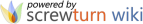(for FireFox 3+ and IE 7+) Contact LAMDA: (email) contact@lamda.nju.edu.cn (tel) +86-025-89681608 © LAMDA, 2019